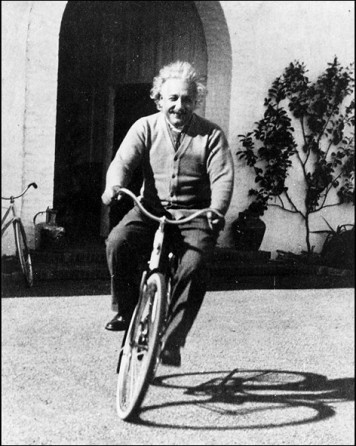# Quantum Control group## PHYS 2006 Classical Mechanics

 Unit Coordinator: Semester: Dr Tim Freegarde 1 Prerequisites: First year physics core units Credit Points: 15 This is a core unit for all physics programmes#### Introduction

This course extends the methods and concepts of Newtonian mechanics introduced in PHYS2015 Motion & Relativity, and provides links to courses on oscillations and waves, quantum mechanics and condensed matter. Beginning with the application of Newton's laws to systems of particles, it moves on to rotational motion, dynamical gravity (Kepler's laws), motion in non-inertial reference frames and systems of coupled oscillators, establishing throughout a rigorous mathematical approach to reinforce and allow analysis of intuitive and everyday situations.

#### Learning Outcomes

After studying this course, students should be able to:

• discuss the linear motion of systems of particles (eg rocket motion)
• define angular momentum for a particle and a system
• define moment of inertia and use it in simple problems
• describe how steady precession occurs and work out the precession rate
• demonstrate that a spherically symmetric object acts gravitationally like a point with the same total mass located at the object's centre (providing you are outside the object)
• solve orbit problems using the conservation of angular momentum and total energy
• explain the origin of the Coriolis and centrifugal terms in the equation of motion in a rotating frame and solve problems in rotating frames
• identify normal modes for oscillating systems
• find normal modes for systems with many degrees of freedom by applying symmetry arguments and boundary conditions

#### Syllabus

1. Linear motion of systems of particles: centre of mass; total external force equals rate of change of total momentum (internal forces cancel); examples (rocket motion)
2. Angular motion: rotations, infinitesimal rotations, angular velocity vector; angular momentum, torque; angular momentum for a system of particles; internal torques cancel for central internal forces; rigid bodies, rotation about a fixed axis, moment of inertia, parallel and perpendicular axis theorems, inertia tensor; precession at steady rate, gyrocompass
3. Gravitation and Kepler's laws: conservative forces; gravity; law of universal gravitation; gravitational attraction of spherically symmetric objects; two-body problem, reduced mass, motion relative to centre of mass; orbits, Kepler's laws; energy considerations, effective potential
4. Non-inertial reference frames: fictitious forces, motion in a frame rotating about a fixed axis, centrifugal and Coriolis terms - apparent gravity, Coriolis deflection, Foucault's pendulum, weather patterns
5. Normal modes: coupled oscillators, normal modes; boundary conditions and Eigenfrequencies

#### Teaching and Learning Methods

Teaching is through a course of about 30 lectures, supplemented by exercises which are addressed weekly in separate problem classes.

#### Non-contact Hours

Students are expected to pursue six hours of independent study per week.

#### Assessment Methods

Weekly exercise sheets comprise 20% of the available marks for this course, with the remaining 80% allocated to the written examination at the end of the semester. Section A of the exam paper will comprise five short, compulsory questions; section B will contain four longer questions, of which only two should be answered.

#### Recommended Books and Course Materials

• G. R. Fowles & G. I. Cassiday - Analytical Mechanics, 7th edition, Brooks/Cole (2005) ISBN 978 0534 408138
• T. L. Chow - Classical Mechanics, 2nd edition, CRC Press (2013) ISBN 978 1466 569960
• A. P. French & M. G. Ebison - Introduction to Classical Mechanics, Springer (1986) ISBN 978 0412 381409
• T. W. Kibble & F. H. Berkshire - Classical Mechanics, 5th edition, Imperial College Press (2004) ISBN 978 1860 944352
• S. T. Thornton & J. B. Marion - Classical Dynamics of Particles and Systems, 5th edition, Brooks/Cole (2003) ISBN 978 8131 518472
• D. Acheson - From Calculus to Chaos - an introduction to dynamics, O U P, Oxford (1998) ISBN 978 0198 500773
• D. Morin - Introduction to Classical Mechanics, C U P, Cambridge (2008) ISBN 978 0521 876223
• L. D. Landau & E. M. Lifshitz - Mechanics, 3rd edition, Butterworth-Heinemann(1976) ISBN 978 0750 628969
• M. L. Boas - Mathematical Methods in the Physical Sciences, Wiley, New York (1983) ISBN 978 0 471 19826 0
• R. P. Feynman- Lectures in Physics, vol. 1, Basic Books (revised 2011) ISBN 978 0 465 02493 3

Lecture notes:
preface

Lecture slides:
set 1  PDF

Problem sheets:
Sheet 1

Examinations:
2010 / solns
2011 / solns
2012 / solns
2013 / solns
2014 / solns
2015 / solns
2016 / solns
report
2017 / solns
report
2018 / solns
report
2019 / solns
report
2020 / solns
report